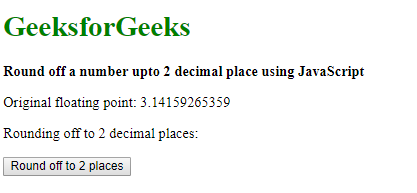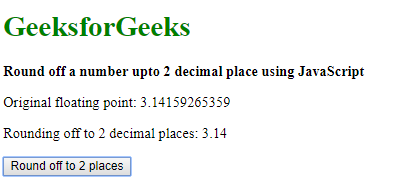# Round off a number upto 2 decimal place using JavaScript

A number can be rounded off to 2 decimal places using 2 approaches:

Method 1: Using the toFixed() method:
The toFixed() method is used to return a string representation of a number up to a certain number of digits after the decimal point. The number is rounded and the fractional part is padded if necessary to make it the specified length. It takes one parameter, that is the number of digits. This is an integer value between 0 to 20.

The toFixed() method is used with a value of 2 to round off a number upto 2 decimal places.

Syntax:

`rounded_number = number.toFixed(2)`

Example:

 ` ` `<``html``> ` ` `  `<``head``> ` `    ``<``title``> ` `      ``Round off a number upto 2  ` `      ``decimal place using JavaScript ` `  `` ` ` ` ` `  `<``body``> ` `    ``<``h1` `style``=``"color: green"``> ` `      ``GeeksforGeeks ` `  `` ` `    ``<``b``>Round off a number upto 2  ` `      ``decimal place using JavaScript ` `  `` ` `    ``<``p``>Original floating point: 3.14159265359 ` `    ``<``p``> ` `      ``Rounding off to 2 decimal places: <``span` `class``=``"output"``> ` `      `` ` `  `` ` ` `  `    ``<``button` `onclick``=``"roundOff()"``> ` `      ``Round off to 2 places ` `  `` ` `    ``<``script` `type``=``"text/javascript"``> ` `        ``function roundOff() { ` `            ``pi = 3.14159265359; ` ` `  `            ``twoPlaces = pi.toFixed(2); ` ` `  `            ``document.querySelector( ` `              ``'.output').textContent = twoPlaces; ` `        ``} ` `    `` ` ` ` ` `  ` `

Output:

• Before clicking the button:• After clicking the button:Method 2: Using the Math.round() method:
The Math.round() method is used to round a number its nearest integer value. It takes one parameter which is the number to be rounded.

The number is first multiplied by 100 to shift the decimal place so that it does not lose its value during the rounding off. The Math.round() method is applied to this number and the final result is divided by 100. This moves the decimal point back to its place as before. This number is the final rounded off number.

Syntax:

`rounded_number = Math.round(number * 100) / 100`

Example:

 ` ` `<``html``> ` ` `  `<``head``> ` `    ``<``title``> ` `      ``Round off a number upto 2 ` `      ``decimal place using JavaScript ` `  `` ` ` ` ` `  `<``body``> ` `    ``<``h1` `style``=``"color: green"``> ` `      ``GeeksforGeeks ` `  `` ` `    ``<``b``>Round off a number upto 2  ` `      ``decimal place using JavaScript ` `  `` ` `    ``<``p``> ` `      ``Original floating point: 3.14159265359 ` `  `` ` `    ``<``p``>Rounding off to 2 decimal places: <``span` `class``=``"output"``> ` `      `` ` `  `` ` ` `  `    ``<``button` `onclick``=``"roundOff()"``> ` `      ``Round off to 2 places ` `  `` ` `    ``<``script` `type``=``"text/javascript"``> ` `        ``function roundOff() { ` `            ``pi = 3.14159265359; ` ` `  `            ``twoPlaces =  ` `              ``Math.round(pi * 100) / 100; ` ` `  `            ``document.querySelector( ` `              ``'.output').textContent = twoPlaces; ` `        ``} ` `    `` ` ` ` ` `  ` `

Output:

• Before clicking the button:• After clicking the button:My Personal Notes arrow_drop_upIm listening

If you like GeeksforGeeks and would like to contribute, you can also write an article using contribute.geeksforgeeks.org or mail your article to contribute@geeksforgeeks.org. See your article appearing on the GeeksforGeeks main page and help other Geeks.

Please Improve this article if you find anything incorrect by clicking on the "Improve Article" button below.